#### Chapter 16 Circles R.D. Sharma Solutions for Class 9th Exercise 16.4

Exercise 16.4

1. In Fig. 16.120, O is the centre of the circle. If ∠APB∠APB= 50°, find ∠AOB and ∠OAB.Solution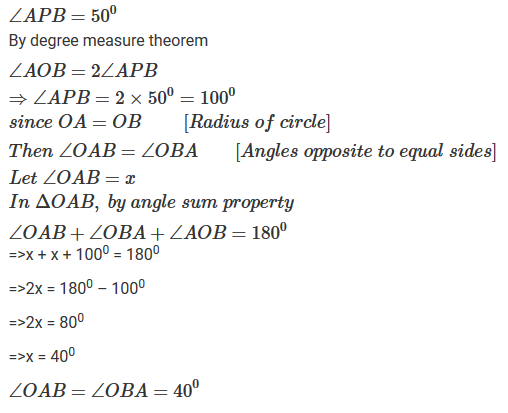2. In Fig. 16.121, it is given that O is the centre of the circle and ∠AOC = 150°. Find ∠ABC.Solution3. In Fig. 16.122, O is the centre of the circle. Find ∠BAC.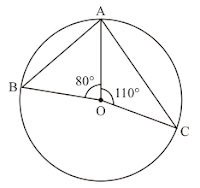Solution4. If O is the centre of the circle, find the value of x in each of the following figures.Solution

(i)(ii)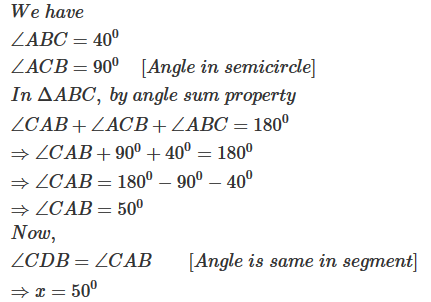(iii)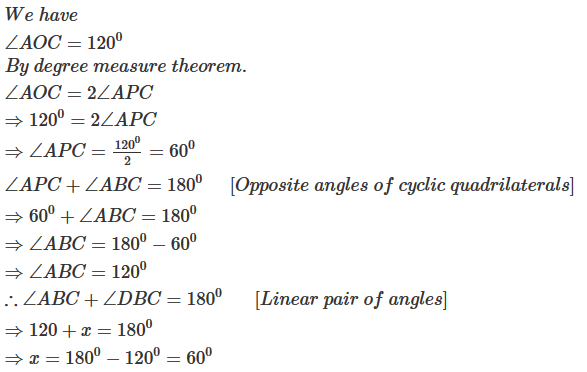(iv)(v)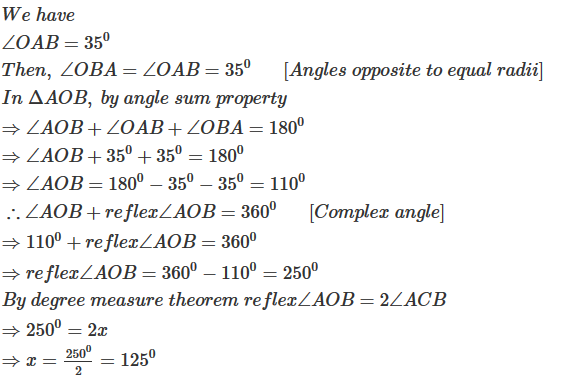(vi)(vii)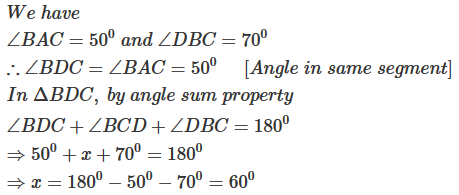(viii)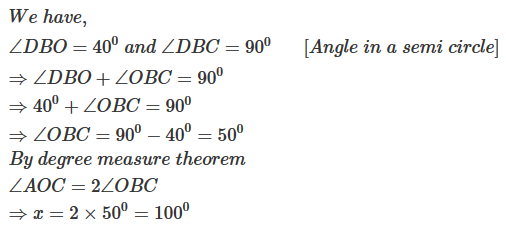(ix)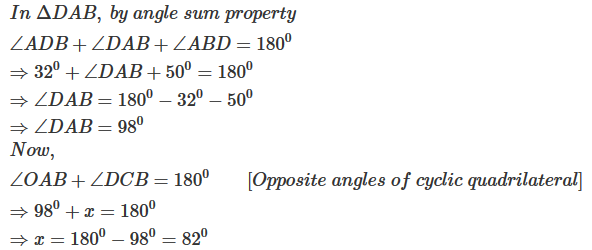(x)(xi)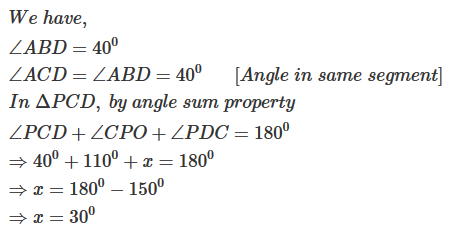(xii)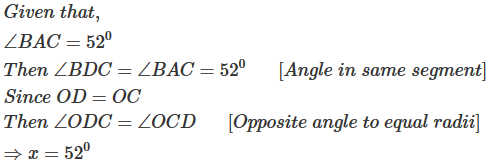5. O is the circumcentre of the triangle ABC and OD is perpendicular on BC. Prove that ∠BOD = ∠A

Solution6. In Fig. 16.135, O is the centre of the circle, BO is the bisector of ∠ABC. Show that AB = AC.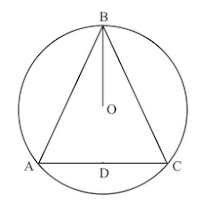Solution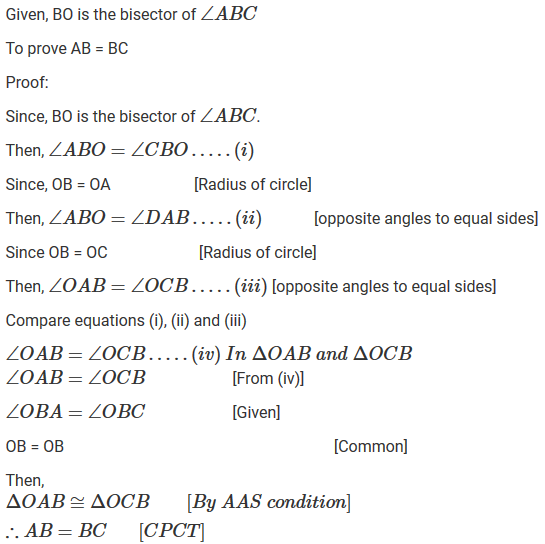7. In Fig. 16.136, O is the centre of the circle, prove that ∠x = ∠y + ∠z.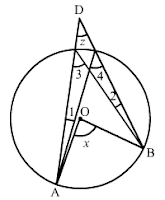Solution8. In Fig. 16.137, O and O' are centres of two circles intersecting at B and C. ACD is a straight line, find x.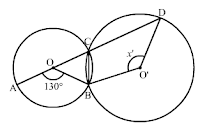Solution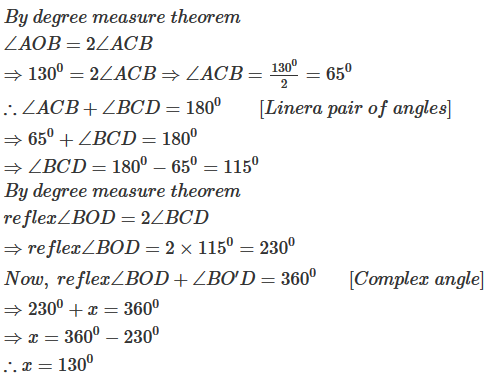9. In Fig. 16.138, O is the centre of a circle and PQ is a diameter. If ∠ROS = 40°, find ∠RTS.Solution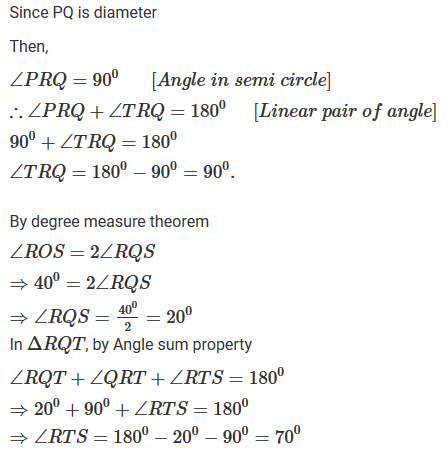10. In Fig. 16.139, if ∠ACB = 40°, ∠DPB = 120°, find ∠CBD.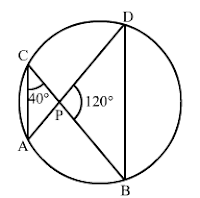Solution11. A chord of a circle is equal to the radius of the circle. Find the angle subtended by the chord at a point on the minor arc and also at a point on the major arc.

Solution